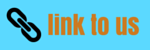# Theorems of Moment of Inertia

## (6) Theorems of Moment of Inertia

• There are two general theorems which proved themselves to be of great importance on moment of inertia
• These enable us to determine moment of inertia of a body about an axis if moment of inertia of body about some other axis is known
i) Perpendicular axis theorem
• This theorem is applicable only to the plane laminar bodies
• This theorem states that, the moment of inertia of a plane laminar about an axis perpendicular to its plane is equal to the sum of the moment of inertia of the lamina about two axis mutually perpendicular to each other in its plane and intersecting each other at the point where perpendicular axis passes through it
• Consider plane laminar body of arbitrary shape lying in the x-y plane as shown below in the figure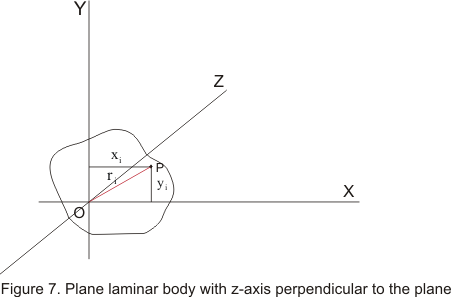• The moment of inertia about the z-axis equals to the sum of the moments of inertia about the x-axis and y axis
• To prove it consider the moment of inertia about x-axis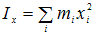where sum is taken over all the element of the mass mi
• The moment of inertia about the y axis is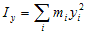• Moment of inertia about z axis is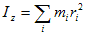where ri is perpendicular distance of particle at point P from the OZ axis
• For each element
ri2=xi2 + yi2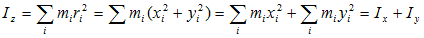ii) Parallel axis theorem
• This theorem relates the moment of inertia about an axis through the center of mass of a body about a second parallel axis
• Let Icm be the moment of inertia about an axis through center of mass of the body and I be that about a parallel axis at a distance r from C as shown below in the figure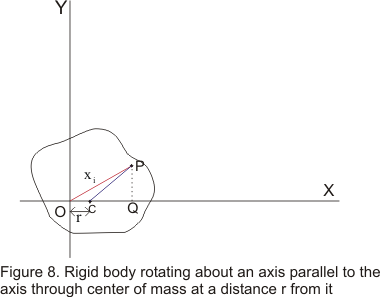Then according to parallel axis theorem
I=Icm+Mr2 where M is the total mass of the body
• Consider a point P of the body of mass mi at a distance xi from O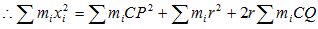• From point P drop a perpendicular PQ on to the OC and join PC.So that
OP2=CP2+ OC2+ 2OC.CQ ( From geometry) and miOP2=miCP2+ miOC2+ 2miOC.CQ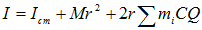• Since the body always balances about an axis passing through center of mass, so algebraic sum of the moment of the weight of individual particles about center of mass must be zero. Here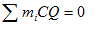which is the algebraic sum of such moments about C and therefore eq as g is constant
Thus we have I=Icm + Mr2 ---(17)
Watch this tutorial for more information on Moment of Inertia